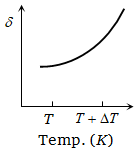A gas mixture consists of 2 mole of oxygen and 4 mole of argon at temperature T. Neglecting all vibrational modes, the total internal energy of the system is

1.  4 RT

2.  15 RT

3.  9 RT

4.  11 RT

High Yielding Test Series + Question Bank - NEET 2020

Difficulty Level:

A jar contains a gas and few drops of water at T K. The pressure in the jar is 830 mm of mercury. The temperature of jar is reduced by 1%. The saturated vapour pressure of water at the two temperatures are 30 mm and 25 mm of mercury. Then the new pressure in the jar will be

1.  917 mm of Hg

2.  717 mm of Hg

3.  817 mm of Hg

4.  None of these

Concept Questions :-

Ideal gas
High Yielding Test Series + Question Bank - NEET 2020

Difficulty Level:

Molar specific heat of oxygen at constant pressure  At constant volume, 5 mol of oxygen is heated from  the quantity of heat required is approximately

1.

2.

3.

4.

High Yielding Test Series + Question Bank - NEET 2020

Difficulty Level:

One mole of an ideal gas requires 207 J heat to raise the temperature by 10 K when heated at constant pressure. If the same gas is heated at constant volume to raise the temperature by the same 10 K, the heat required is

1.  198.7 J

2.  29 J

3.  215.3 J

4.  124 J

(Given the gas constant )

High Yielding Test Series + Question Bank - NEET 2020

Difficulty Level:

The expansion of an ideal gas of mass m at a constant pressure P is given by the straight line D. Then the expansion of the same ideal gas of mass 2m at a pressure P/ 2 is given by the straight line , where number on graphs indicate slope , is-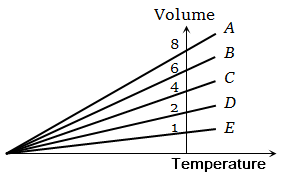1.  E

2.  C

3.  B

4.  A

High Yielding Test Series + Question Bank - NEET 2020

Difficulty Level:

An experiment is carried on a fixed amount of gas at different temperatures and at high pressure such that it deviates from the ideal gas behaviour. The variation of $\frac{\mathrm{PV}}{\mathrm{RT}}$ with P is shown in the diagram. The correct variation will correspond to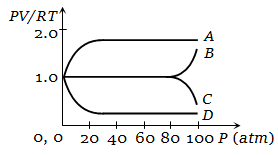1. Curve A

2. Curve B

3. Curve C

4. Curve D

High Yielding Test Series + Question Bank - NEET 2020

Difficulty Level:

The graph which represent the variation of mean kinetic energy of molecules with temperature t°C is

1.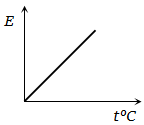2.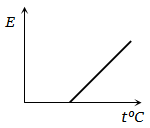3.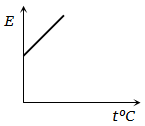4.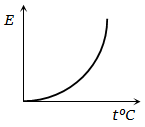High Yielding Test Series + Question Bank - NEET 2020

Difficulty Level:

The adjoining figure shows graph of pressure and volume of a gas at two temperatures ${\mathrm{T}}_{1}$ and ${\mathrm{T}}_{2}$. Which of the following interferences is correct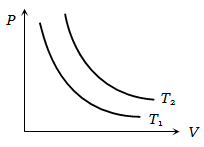1.  ${\mathrm{T}}_{1}>{\mathrm{T}}_{2}$

2. ${\mathrm{T}}_{1}={\mathrm{T}}_{2}$

3. ${\mathrm{T}}_{1}<{\mathrm{T}}_{2}$

4. No interference can be drawn

High Yielding Test Series + Question Bank - NEET 2020

Difficulty Level:

The expansion of until mass of a perfect gas at constant pressure is shown in the diagram. Here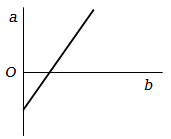1. a = volume, b = $°\mathrm{C}$ temperature

2. a = volume, b = $\mathrm{K}$ temperature

3. a = $°\mathrm{C}$ temperature, b = volume

4. a = $\mathrm{K}$ temperature, b = volume

High Yielding Test Series + Question Bank - NEET 2020

Difficulty Level:

An ideal gas is initially at temperature T and volume V. Its volume is increased by $∆\mathrm{V}$ due to an increase in temperature $∆\mathrm{T}$, pressure remaining constant. The quantity $\mathrm{\delta }=∆\mathrm{V}/\left(\mathrm{V}∆\mathrm{T}\right)$ varies with temperature as

1.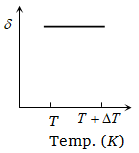2.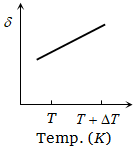3.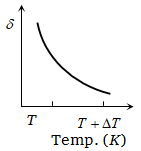4.